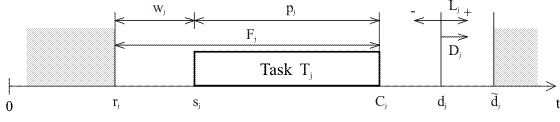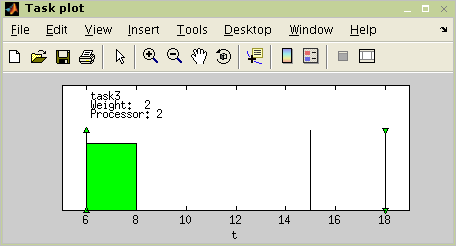# TORSCHE Scheduling Toolbox for Matlab

### 1. Introduction

Task is a basic term in scheduling problems describing a unit of work to be scheduled. The terminology is adopted from the following publications: I – [Błażewicz01], II – [Butazo97], III – [Liu00]. Graphic representation of task parameters is shown in Figure 3.1, “Graphics representation of task parameters”. Task `T`j in the toolbox is described by the following properties:

Name (`Name`)

Processing timeI pj (`ProcTime`)

is the time necessary to execute task `T`j on the processor without interruption

(Computation time II)

Release timeIII rj (`ReleaseTime`)

(Arrival time I,II, Ready time I, Request time II)

DeadlineI dj (`Deadline`)

specifies a time limit by which the task has to be completed, otherwise the scheduling is assumed to fail

Due dateI d~j (`DueDate`)

specifies a time limit by which the task should be completed, otherwise the criterion function is charged by penalty

WeightI (`Weight`)

expresses the priority of the task with respect to other tasks (Priority II)

Processor (`Processor`)

specifies dedicated processor on which the task must be executedFigure 3.1. Graphics representation of task parameters

Rest of the task properties shown in Figure 3.1, “Graphics representation of task parameters” are related to start time of task `s`j, i.e. result of scheduling (see sections Section 4.1, “Start Time of Task” and Section 3.2, “Schedule”). Properties completion time `C`j (`C`j=`s`j+`p`j), waiting time `w`j (`w`j=`s`j+`r`j), flow time `F`j (`F`j=`C`j-`r`j), lateness `L`j (Lj=`C`j+`d`j) and tardiness `D`j (`D`j=`max``{C`j-dj,0}) can be derived from start time `s`j.

### 2. Creating the `task` Object

In the toolbox, task is represented by the object `task`. This object is created by the command with the following syntax rule (properties contained inside the square brackets are optional):

```t1 = task([Name,]ProcTime[,ReleaseTime[,Deadline[,DueDate
[,Weight[,Processor]]]]])```

Command `task` is a constructor of object `task` and returns the object. In the syntax rule above the object is the variable `t1`. Examples of the object task creating are shown in Figure 3.2, “Creating task objects”.

```>> t1 = task(5)
Processing time: 5
Release time:    0
Processing time: 5
Release time:    3
Processing time: 2
Release time:    6
Due date:        15
Weight:          2
Processor:       2```

### 3. Graphical Representation of the `task` Object

Parameters of a task can be graphically displayed using command `plot`. For example parameters of task `t3`, created above, can be displayed by command:

`>> plot(t3)`

For more details see Reference Guide @task/plot.m.Figure 3.3. Plot example

### 4. Object `task` Modifications

Command `get` returns the value of the specified property or values of all properties. Command `set` sets the value of the specified property. These two commands has the same syntax as is described in Matlab user's guide. Property access is allowed using the . (dot) operator too.

Note

To obtain a list of all accessible properties use command `get`. Note that some private and virtual properties aren't accessible using the . (dot) operator, although they are presented when the automatic completion by Tab key is used.

```>> get(t3)
ProcTime: 2
ReleaseTime: 6
DueDate: 15
Weight: 2
Processor: 2
UserParam:
Notes: ''
>> set(t3,'ProcTime',4)
>> get(t3,'ProcTime')

ans =

4```

#### 4.1. Start Time of Task

Command `add_scht` adds the start time into an object task. Schedule of a task is described by three arrays (`start`, `length`, `processor`). The length of array is equal to number of task preemptions minus one. Opposite command to ```get_scht is appointed for getting a schedule from the task object. ```For more details see Reference Guide @task/add_scht.m , @task/get_scht.m.

#### 4.2. Color Modification

Commands `set_graphic_param` and `get_graphic_param` can be used to define color of tasks. If color of task is set, command `plot` will use it. Use of these commands is shoved on the following example:

```>> t = task('task',5);
>> set_graphic_param(t,'color','red')
>> get_graphic_param(t,'color')

ans =

red```

Periodic tasks are tasks, which are released periodically with a fixed period. There is a `ptask` object in TORSCHE™ that allows users to work with periodic tasks. Periodic tasks are mainly used in real-time scheduling area (see Chapter 8, Real-Time Scheduling).

#### 5.1. Creating the `ptask` Object

The syntax of `ptask` constructor is:

```pt = ptask([Name,]ProcTime,Period[,ReleaseTime[,Deadline[,Duedate[,Weight[,Processor]]]]])
```

Almost all parameters are the same as for `task` object except for `Period`, which specifies the period of the task.

#### 5.2. Working with `ptask` Objects

The way of manipulating `ptask` objects is the same as for `task` objects. It is possible to change their properties using `set` and `get` methods as well as by dot notation. In addition, there is `util` method which returns CPU utilization factor of the task.

Webmaster - Jan Dvořák (2004 - 2020)